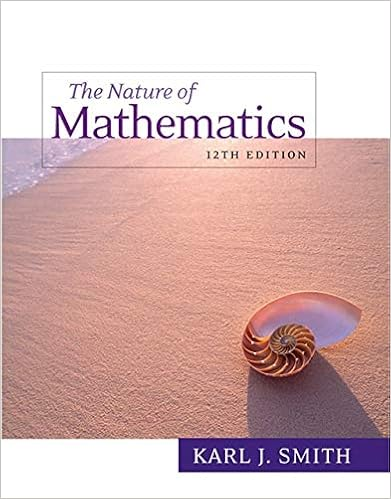# MATH 107 Final Exam.pdf - College Algebra MATH 107 Spring...

• Test Prep
• 11
• 54% (37) 20 out of 37 people found this document helpful

This preview shows page 1 - 3 out of 11 pages.

##### We have textbook solutions for you!
The document you are viewing contains questions related to this textbook.The document you are viewing contains questions related to this textbook.
Chapter 3 / Exercise 33
Nature of Mathematics
SmithExpert Verified
College Algebra MATH 107 Spring, 2019, V1.6 Page 1 of 11 MATH 107 FINAL EXAMINATION This is an open-book exam. You may refer to your text and other course materials as you work on the exam, and you may use a calculator. You must complete the exam individually. Neither collaboration nor consultation with others is allowed.Record your answers and work on the separate answer sheet provided. There are 30 problems. Problems #112 are Multiple Choice. Problems #1321 are Short Answer. (Work not required to be shown) Problems #2230 are Short Answer with work required to be shown. MULTIPLE CHOICE 1. Determine the domain and range of the piecewise function. 1. ______ A. Domain [3, 3]; Range [2, 4] B. Domain [2, 4]; Range [3, 3] C. Domain [2, 2]; Range [1, 4] D. Domain [2, 3/2]; Range [3/2, 4] 2. Solve: 173xx+=2. ______ A. No solution B. 1 C. 7 D. 1, 8 -2 2 4 -4 -2 -4 2 4
##### We have textbook solutions for you!
The document you are viewing contains questions related to this textbook.The document you are viewing contains questions related to this textbook.
Chapter 3 / Exercise 33
Nature of Mathematics
SmithExpert Verified
College Algebra MATH 107 Spring, 2019, V1.6 Page 2 of 11 3. Determine the interval(s) on which the function is decreasing.3. ______
1)
)
2)
) 4. Determine whether the graph of 7yx=+is symmetric with respect to the origin, the x-axis, or the y-axis. 4. ______
•••